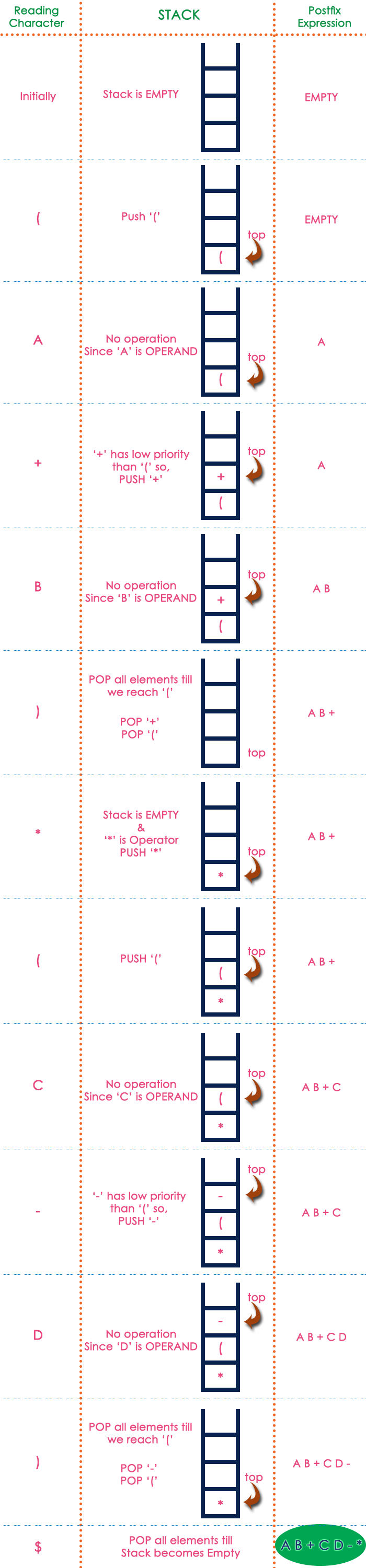The perfect place for easy learning...

×

# Infix to Postfix Conversion

Any expression can be represented using three types of expressions (Infix, Postfix, and Prefix). We can also convert one type of expression to another type of expression like Infix to Postfix, Infix to Prefix, Postfix to Prefix and vice versa.

To convert any Infix expression into Postfix or Prefix expression we can use the following procedure...

1. Find all the operators in the given Infix Expression.
2. Find the order of operators evaluated according to their Operator precedence.
3. Convert each operator into required type of expression (Postfix or Prefix) in the same order.

## Example

Consider the following Infix Expression to be converted into Postfix Expression...

#### D = A + B * C

• Step 1 - The Operators in the given Infix Expression : = , + , *
• Step 2 - The Order of Operators according to their preference : * , + , =
• Step 3 - Now, convert the first operator * ----- D = A + B C *
• Step 4 - Convert the next operator + ----- D = A BC* +
• Step 5 - Convert the next operator = ----- D ABC*+ =

Finally, given Infix Expression is converted into Postfix Expression as follows...

# Infix to Postfix Conversion using Stack Data Structure

To convert Infix Expression into Postfix Expression using a stack data structure, We can use the following steps...

1. Read all the symbols one by one from left to right in the given Infix Expression.
2. If the reading symbol is operand, then directly print it to the result (Output).
3. If the reading symbol is left parenthesis '(', then Push it on to the Stack.
4. If the reading symbol is right parenthesis ')', then Pop all the contents of stack until respective left parenthesis is poped and print each poped symbol to the result.
5. If the reading symbol is operator (+ , - , * , / etc.,), then Push it on to the Stack. However, first pop the operators which are already on the stack that have higher or equal precedence than current operator and print them to the result.

### Example

Consider the following Infix Expression...

#### ( A + B ) * ( C - D )

The given infix expression can be converted into postfix expression using Stack data Structure as follows...The final Postfix Expression is as follows...